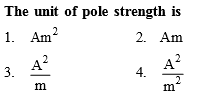You’ve reached the end of your free Videos limit.
#6 | Torque Acting on Bar Magnet in Uniform Field
(Physics) > Magnetism and Matter
Related Practice Questions :Complete Question Bank + Test Series
Complete Question Bank

Difficulty Level:

The magnetic field at a point x on the axis of a small bar magnet is equal to the field at a point y on the equator of the same magnet. The ratio of the distances of x and y from the centre of the magnet is
(a) ${2}^{-3}$

(b) ${2}^{-1}{3}}$

(c) ${2}^{3}$

(d) ${2}^{1}{3}}$

Complete Question Bank + Test Series
Complete Question Bank

Difficulty Level:

Points A and B are situated perpendicular to the axis of a 2cm long bar magnet at large distances X and 3X from its centre on opposite sides. The ratio of the magnetic fields at A and B will be approximately equal to
(a) 1 : 9               (b) 2 : 9
(c) 27 : 1             (d) 9 : 1

Complete Question Bank + Test Series
Complete Question Bank

Difficulty Level:

Two short magnets with their axes horizontal  to the magnetic meridian are placed with their centres 40 cm east and 50 cm west of magnetic needle. If the needle remains undeflected, the ratio of their magnetic moments  is

(a) 4:5

(b) 16:25

(c) 64:125

(D) 2:$\sqrt{5}$

Complete Question Bank + Test Series
Complete Question Bank

Difficulty Level:

If a bar magnet of magnetic moment M is freely suspended in a uniform magnetic field of strength B, the work done in rotating the magnet through an angle is

(a) $MB\left(1-\mathrm{sin}\theta \right)$

(b) $MB\mathrm{sin}\theta$

(c) $MB\mathrm{cos}\theta$

(d) $MB\left(1-\mathrm{cos}\theta \right)$